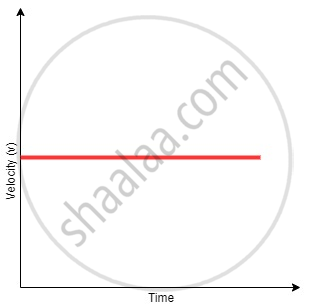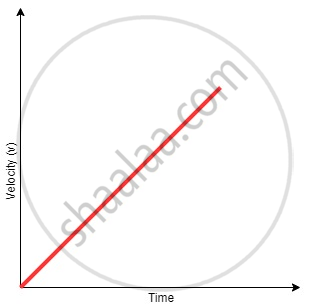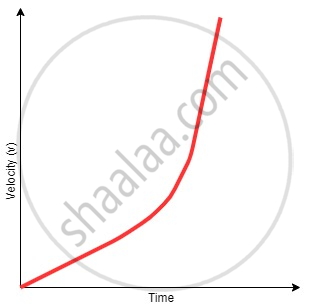# Graphical Representation of Linear Motion - Velocity - Time Graphs

#### notes

Velocity-Time Graphs:

To draw velocity time graphs, we will use the three equations of motion.

Case 1: Velocity-time graphs with constant velocity (zero acceleration)

When the velocity is constant, the velocity time graph, with Y-axis denoting velocity and X-axis denoting time, will be like:As clear from the graph, the velocity is constant (c) throughout the time interval. No particles of matter how much the time changes, the velocity will be c at every instant. In this case, we have taken the initial velocity to be positive. The graph will be different if the initial velocity is negative.

Example: If the acceleration of a particle is zero (0), and velocity is constantly say 5 m/s at t =0, then it will remain constant throughout the time.

Case 2: Velocity-time graphs with constant acceleration

When the acceleration is constant (positive), and the initial velocity of the particle is zero, the velocity of the particle will increase linearly as predicted by the equation:

v = u + at

Since u = 0

v = atAs shown in the figure, the velocity of the particle will increase linearly with respect to time. The slope of the graph will give the magnitude of acceleration.

Example: If the acceleration of a particle is constant (k) and is positive, the initial velocity is zero, and then the velocity increases linearly. The slope of the velocity-time graph will give the acceleration.

Case 3: Velocity-time graphs with increasing acceleration

When the acceleration is increasing with time, the velocity-time graph will be a curve as predicted from the equation:
v = u + at
Since u = 0
v= atSince acceleration is a function of time, velocity-time graph will be a curve.
Note: Since the acceleration is continuously increasing with time, the magnitude of the slope will also continuously increase with time.

When an object moves along a straight line with uniform acceleration, it is possible to relate its velocity, acceleration during motion and the distance covered by it in a certain time interval by a set of equations known as the equations of motion. For convenience, a set of three such equations are given below:
v = u + at                          ......(1)
s = "ut" + 1/2at^2                  ......(2)
2 as = "v"^2 – "u"^2                   ......(3)

where u is the initial velocity of the object which moves with uniform acceleration a for time t, v is the final velocity, and s is the distance travelled by the object in time t. Eq.(1) describes the velocity-time relation and Eq.(2) represents the position-time relation.

Eq. (3), which represents the relation between the position and the velocity, can be obtained from Eqs.(1) and (2) by eliminating t.

These three equations can be derived by graphical method.

If you would like to contribute notes or other learning material, please submit them using the button below.

### Shaalaa.com

Velocity Time Graph [00:23:17]
S
0%